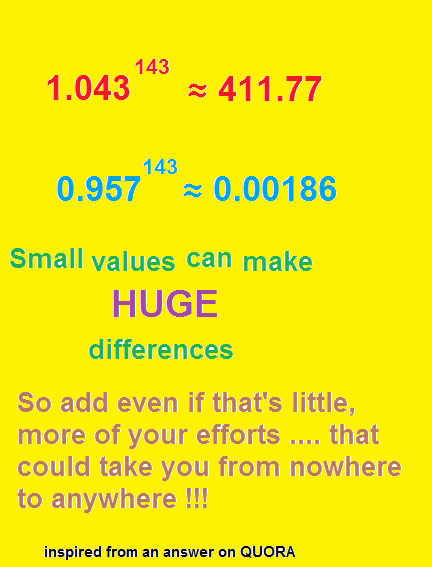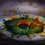# Don't miss anything...This is not about any technique of problem solving but i found it too inspiring, even that gives a message about $$\color{Red}{\text{taking}}$$ $$\color{Red}{\text{up}}$$ $$\color{Red}{\text{challenges}}$$ , including $$\color{Red}{\text{solving}}$$ $$\color{Red}{\text{various}}$$ $$\color{Red}{ \text{problems}}$$....

See ! The two numbers just differ by 0.086 and see what happened to the results ! You efforts are like this only , adding little more everyday could make you from a very beginner to an expert....

$\color{#69047E}{\text{Keep}}$ $\color{#69047E}{\text{trying}}$, even a small amount of information, like the $\color{#20A900}{\text{unidentified}}$ $\color{#20A900}{\text{quadrilaterals}}$ (cyclic mostly) in $\color{limegreen}{\text{Geometry}}$, or some $\color{#20A900}{\text{tricks}}$ in $\color{limegreen}{\text{number}}$ $\color{limegreen}{\text{theory}}$ problems, some $\color{#20A900}{\text{useful}}$ $\color{#20A900}{\text{substitutions}}$ in $\color{limegreen}{\text{Algebra}}$ and $\color{limegreen}{\text{Calculus}}$, simple things like $\color{#20A900}{\text{units}}$ in $\color{limegreen}{\text{Physics}}$ (yeah, that makes a huge difference if you consider wrong units)..... anything !

Everything is useful and small things make $\color{#3D99F6}{Huge}$ differences ! Don't miss anything when you work on a problem, especially the olympiad type !

Good luck Problem Solving....7 years, 1 month ago

This discussion board is a place to discuss our Daily Challenges and the math and science related to those challenges. Explanations are more than just a solution — they should explain the steps and thinking strategies that you used to obtain the solution. Comments should further the discussion of math and science.

When posting on Brilliant:

• Use the emojis to react to an explanation, whether you're congratulating a job well done , or just really confused .
• Ask specific questions about the challenge or the steps in somebody's explanation. Well-posed questions can add a lot to the discussion, but posting "I don't understand!" doesn't help anyone.
• Try to contribute something new to the discussion, whether it is an extension, generalization or other idea related to the challenge.

MarkdownAppears as
*italics* or _italics_ italics
**bold** or __bold__ bold
- bulleted- list
• bulleted
• list
1. numbered2. list
1. numbered
2. list
Note: you must add a full line of space before and after lists for them to show up correctly
paragraph 1paragraph 2

paragraph 1

paragraph 2

[example link](https://brilliant.org)example link
> This is a quote
This is a quote
    # I indented these lines
# 4 spaces, and now they show
# up as a code block.

print "hello world"
# I indented these lines
# 4 spaces, and now they show
# up as a code block.

print "hello world"
MathAppears as
Remember to wrap math in $$ ... $$ or $ ... $ to ensure proper formatting.
2 \times 3 $2 \times 3$
2^{34} $2^{34}$
a_{i-1} $a_{i-1}$
\frac{2}{3} $\frac{2}{3}$
\sqrt{2} $\sqrt{2}$
\sum_{i=1}^3 $\sum_{i=1}^3$
\sin \theta $\sin \theta$
\boxed{123} $\boxed{123}$

Sort by:

Good words of a person...

- 7 years, 1 month ago

Exercise: Find what's wrong with this logic.

- 7 years, 1 month ago

Any number larger than 1 will increase when you raise it to a higher power and numbers $\leq 1$ will not,so you could do this with 1.0000000001 and 0.999999999999 :)

- 7 years, 1 month ago

Inspirational

- 7 years, 1 month ago

true inspiration...!!!!

- 7 years, 1 month ago

Yeah... You put the message very nicely decorated with a mathematical proof.

- 6 years, 10 months ago

Just because it is possible for a small thing to make a huge difference doesn't mean that it is likely. There are far more small things than huge differences for them to make.

- 6 years, 10 months ago